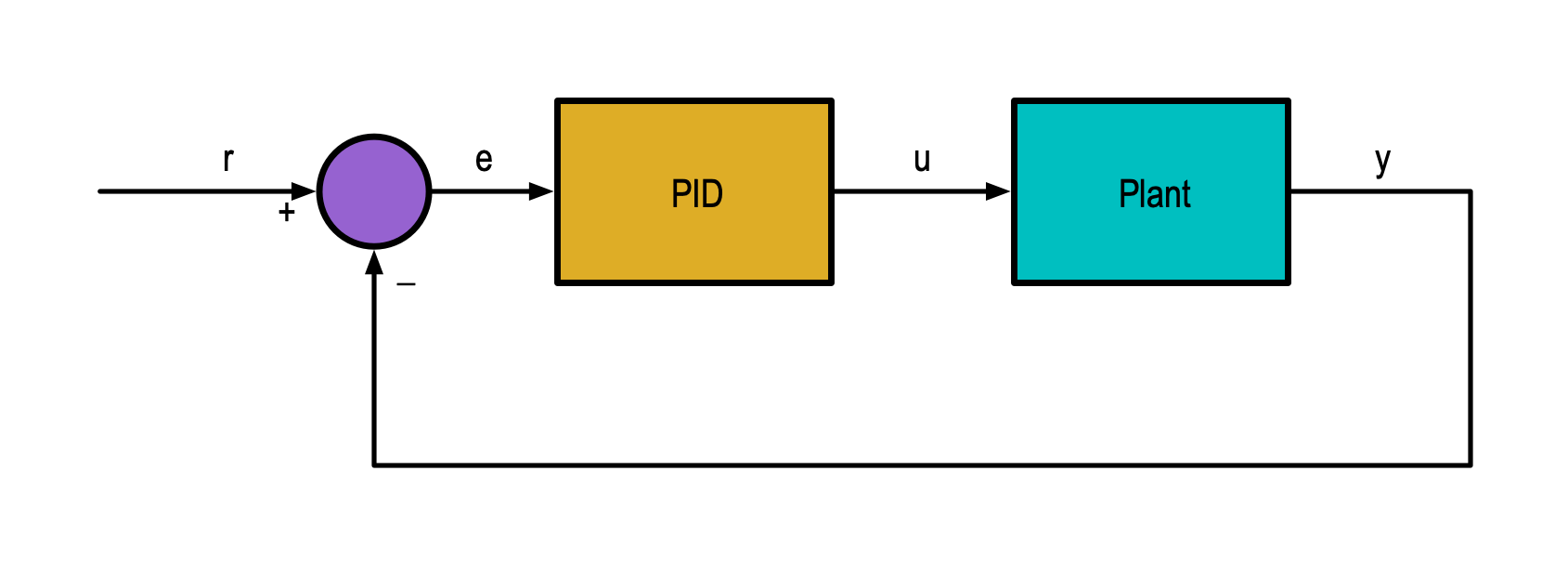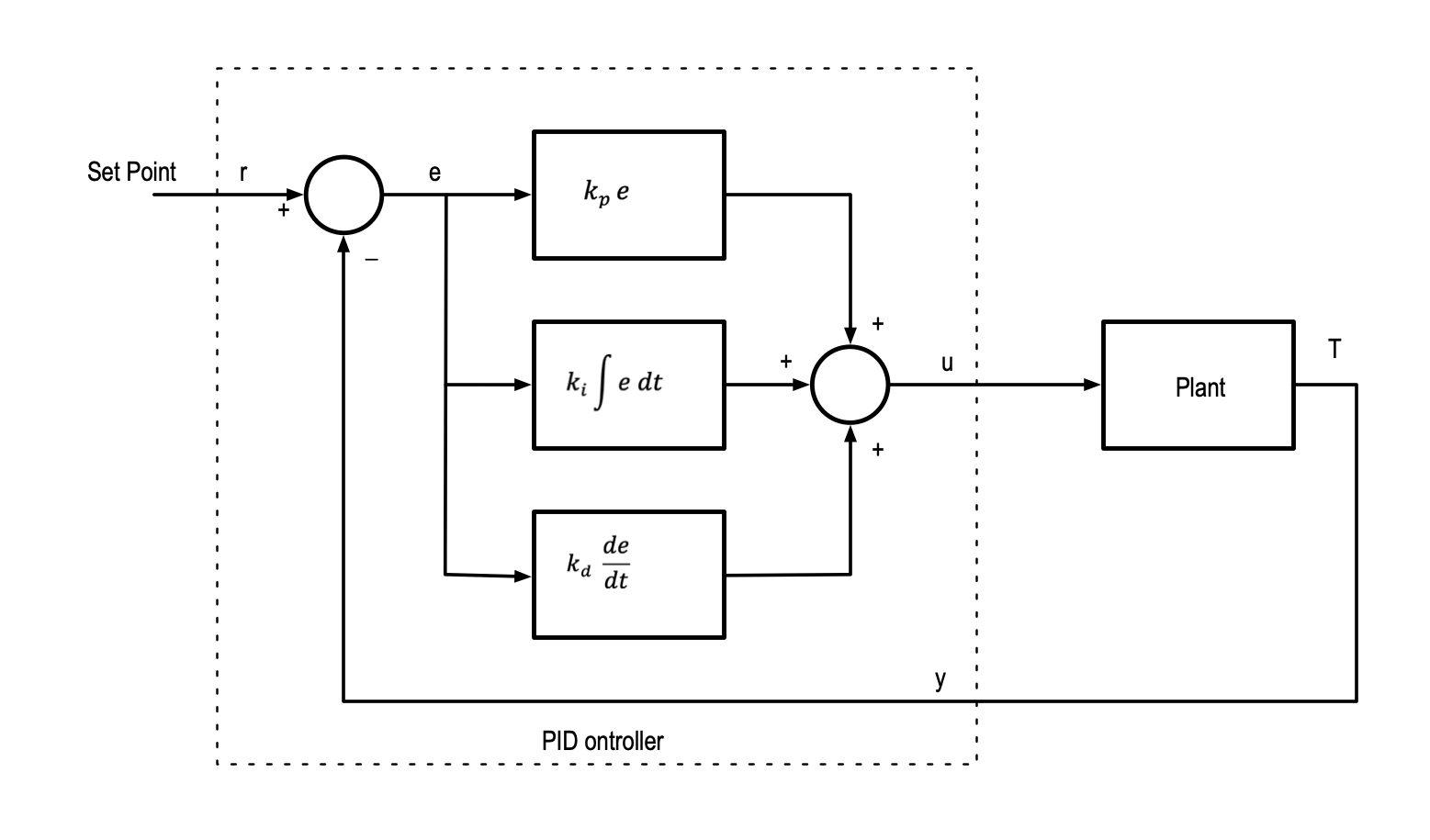# PID ControlWritten by

PID (Proportional, Integral, Derivative) control is a control technique that is frequently used in closed loop control systems to regulate quantities to a setpoint of some kind. Take for example a temperature-controlled soldering iron—you set a temperature and the controller regulates the current through the heating element to maintain the set temperature at the tip regardless of the thermal load.

Figure 1 shows how we would represent this as a control system. The box marked “Plant” is the power control circuit and the soldering iron itself. The input to this is a control signal indicating how much power we want to apply to the heating element and the output is a signal corresponding to the tip temperature. The controller is inside the dotted box. This includes has two inputs: y representing the temperature feedback and r representing the setpoint. It has one output u which is the control signal to the plant.FIGURE 1. This is the block-diagram representation of our hypothetical closed-loop control system. The box marked “Plant” is the power control circuit and the soldering iron itself. The controller inside the dotted box calculates the error e by subtracting the feedback signal y form the setpoint r. Some control law uses the error signal to calculate the signal u which controls the power to the soldering iron.

The feedback signal is subtracted from the setpoint to create an error signal e that represents the difference between the setpoint temperature and the actual tip temperature. So how do we use this error to create the control signal? Let’s start with the simplest approach and just multiply the error by some constant kp. we call this a proportional gain, since the output u is proportional to the input e.

Now if the soldering iron is at room temperature and we set the setpoint to 300ºC, the error will be a large positive number and therefore the control signal u will also be large (in fact it will probably saturate at full power—a thought we need to keep in mind). The tip temperature will begin to rise and as it approaches the setpoint the error signal will reduce as shown in Figure 2. Note that the tip temperature can never quite reach the setpoint because if it did, the error term will be zero and so the control signal u will be zero. The system reaches a steady state with a small error (if you choose kp right).FIGURE 2. If we use only proportional gain, the temperature can never quite reach the set-point. If it did, the error term would drop to zero and there could be no output u, and thus no drive to the iron. To overcome this, we need to introduce an integral term to the control law. This accumulates the steady state error over time, increasing u until the error is driven to zero. At this point there is nothing to integrate, and the output remains fixed (as long as nothing else changes).

We can address this problem by introducing an integral term into the controller. The integral term just means that the error is accumulated over time (think charging a capacitor). Mathematically we would write:

The constant ki controls how much of the integral term is added to the control signal. The effect of this is to eliminate the steady state error. Imagine the tip temperature is just below the setpoint. The proportional gain can’t move us any further, but the integral term continues to grow with time since it is continually accumulating the error and providing extra power to the heater.

Once the temperature reaches the setpoint, the error is zero, so the proportional gain contributes nothing, and the output signal is purely provided by the integral gain. Since the error is now zero the integrator is effectively frozen as there is no more error to accumulate. (I’ve simplified this explanation a little bit since the integral gain is working all the time, not just when the proportional gain reaches steady state).

Now it takes a bit of time for the temperature to reach the setpoint since the integral term is by nature a low-pass filter. We can add a derivative term to effectively give a “kick” to the control signal if there is a rapid change in setpoint, or a rapid change in tip temperature (say when soldering a joint with high thermal mass). The derivative term is related to the rate of change of the error signal:

The constant kd controls how much of the derivative term is added to the control signal. The effect is to give a “boost” to the output u when there is a rapid change in the error signal. The derivative term becomes less significant as the error stabilizes and is zero when the error not changing.

Figure 3 is the control block diagram of the complete PID controller. This can be implemented in analog circuitry or in the digital domain as is much more common these days. In this case, the setpoint and feedback are digitized and the output is likely to be a PWM signal with duty cycle proportional to the output u.FIGURE 3. The block diagram of the PID controller. The proportional, integral and derivative terms are calculated separately and added together to create the output signal. Selecting the right gains for a given system is a tricky proposition. There are some mathematical and empirical approaches.

This is the theory. In practice there are a few issues to be aware of. The derivative term is a bit dangerous since it has very high gain for high frequency signals which means any noise or spikes on the feedback or setpoint signals can send the output off the scale. In practice we need to add a low-pass filter of some kind to the error before calculating the derivative term. In many control cases the derivative is not required at all.

The integrator can suffer “wind-up” if the output saturates. In this case the error can never be reduced to zero and the integral term will continue to grow even if the output is fixed. Now when the setpoint changes and the integrator has to “unwind” the accumulated error before regulation resumes. This can have nasty side effects. There are “anti-windup” measures that can be taken, but they are beyond the scope of this discussion.

Finally, selecting the right values for the proportional, integral and derivative gains (known as tuning the PID controller) is quite involved, and achieving optimum response with minimum overshoot can take a bit of work. There are some techniques such as the Zeigler-Nichols method and others that can provide a good starting point.

References

Åström, Karl J., and Richard M. Murray. Feedback Systems: An Introduction for Scientists and Engineers. Princeton, NJ: Princeton Univ. Press, 2008.

Dorf, Richard C., and Robert H. Bishop. Modern Control Systems. 12. ed. Upper Saddle River, NJ: Prentice Hall, Pearson, 2011.

“PID Controller.” In Wikipedia, January 29, 2022. https://en.wikipedia.org/w/index.php?title=PID_controller&oldid=1068697253

 Keep up-to-date with our FREE Weekly Newsletter! Don't miss out on upcoming issues of Circuit Cellar. Subscribe to Circuit Cellar Magazine Note: We’ve made the May 2020 issue of Circuit Cellar available as a free sample issue. In it, you’ll find a rich variety of the kinds of articles and information that exemplify a typical issue of the current magazine. Would you like to write for Circuit Cellar? We are always accepting articles/posts from the technical community. Get in touch with us and let's discuss your ideas.